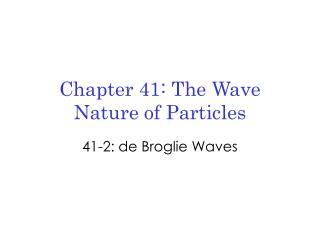DownloadDownload PresentationChapter 41: The Wave Nature of Particles

# Chapter 41: The Wave Nature of Particles

Télécharger la présentation## Chapter 41: The Wave Nature of Particles

- - - - - - - - - - - - - - - - - - - - - - - - - - - E N D - - - - - - - - - - - - - - - - - - - - - - - - - - -
##### Presentation Transcript

1. Chapter 41: The Wave Nature of Particles 41-2: de Broglie Waves

2. Louis-Victor de Broglie (1892-1987) • 1924: predicts wave nature of particles • Photons have wave/particle nature • Symmetry suggests particles should have wave nature • Corpuscular nature of photons gives us E=hf, but f is related to the wave nature of light. The two must be intimately connected.

3. de Broglie Wavelength • Use same relationship as defined by Einstein: • Any particle that has momentum, has a wavelength associated with it. (N.B. v is a speed, not a velocity!)

4. Wave Nature of Particles • Photon/light – wave in electromagnetic field • Matter – probability distribution • Average probability of location of particle

5. Quantum Mechanics • Particles have wave nature associated with their average probability of location • We can’t know *BOTH* a particle’s position in space and its momentum at the same time (more to come on this later…) • Fuzzy matter…

6. Calculating de Broglie wavelength • Need matter that has momentum: person riding a bike. m = 80 kg v = 9 m/sec

7. Activity There is a student who is chasing a school bus. She is going as fast as she can to catch the bus, and the bus is stopping to let her catch up. Given that the mass of the person is 70 kg, her speed is 2 m/sec, the mass of the bus is 3,000 kg, and the speed of the bus is 0.1 m/sec, (a) what is the de Broglie wavelength of the person? (b) What is the de Broglie wavelength of the bus? (c)Which one is bigger? (d)What should change if their de Broglie wavelengths are the same? Make the necessary change! (N.B. the person is running as fast as she can!)

8. Solution m = 70 kg v = 2 m/sec m = 3,000 kg v = 0.1 m/sec

9. Solution (person)

10. Solution (bus)

11. Solution (comparison) The person’s de Broglie wavelength is bigger than the de Broglie wavelength of the bus! The more massive an object is, the smaller its de Broglie wavelength. Since the person is running as fast as she can, the bus must go slower to get their wavelengths to be the same. How fast should the bus be going?

12. Solution (continued)

13. de Broglie Wavelength • These numbers are very small! • Size of atom = 10-10m • So, the de Broglie wavelength of an object is really only interesting when lde Broglie>10-10m. (This is why busses are not “fuzzy”!)

14. What could we change to make the de Broglie wavelength interesting? • h is Planck’s constant (a *very* small number) • v is the speed of the object (usually not very small) • m is the mass of the object • Subatomic particles have *very* small masses!

15. de Broglie wavelengths of subatomic particles • If we decrease the mass enough (10-26 kg), the de Broglie wavelength can be on the order of the size of the atom for a large range of speeds. • For such particles, we should see them behave as waves do: • Electron diffraction (coming soon!)

16. Implications of the wave nature of particles • The Bohr atom • Including the wave nature in the picture of an electron makes the idea of quantized energy levels seem much more natural. • Before: • Angular momentum is quantized (not really intuitive – mass, velocity and radius are not quantized!) • Now: • Standing wave modes explain why electrons exist with discrete energies within an atom (allowed energy levels exist because a standing wave pattern with an integer number of wavelengths can exist at those energies)

17. Minute Paper • What is most clear, because of today’s class? • What is still unclear, because of today’s class?